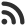Feed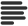Articles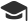Tutorials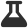CodingLab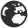CommunityLeaderboardFounder & Programmer at HackersFriend Updated June 6, 2019, 7:12 p.m. ⋅ 53 views

# Length of longest sub-array with maximum arithmetic mean

Problem statement:

Given an array of n-elements find the longest sub-array with the greatest arithmetic mean. The length of the sub-array must be greater than 1 and the mean should be calculated as an integer only.

Example:

``````Input:
arr[] = {3, 2, 1, 2}

Output : 2
sub-array 3, 2 has greatest arithmetic mean

Input :arr[] = {3, 3, 3, 2}
Output : 3``````

Solution Idea:

First find the greatest mean of two consecutive elements from the array. Again iterate over the array and try to find the longest sequence in which each element must be greater or equal to the greatest mean calculated.

Why it works ?

Above approach works because of these key points:

• Minimum possible sequence length is 2 and hence the greatest mean of two consecutive elements will always be part of the result.
• Any element which is equal or greater than the calculated mean may be the part of the longest sequence.

Implementation

``````#include <bits/stdc++.h>
using namespace std;

// Function to find maximum distance between unequal elements
int longestSubarray(int arr[], int n)
{

// Calculate maxMean
int maxMean = 0;
for (int i = 1; i < n; i++)
maxMean = max(maxMean,
(arr[i] + arr[i - 1]) / 2);

// Iterate over array and calculate largest subarray
// with all elements greater or equal to maxMean
int ans = 0;
int subarrayLength = 0;
for (int i = 0; i < n; i++)
if (arr[i] >= maxMean)
ans = max(ans, ++subarrayLength);
else
subarrayLength = 0;

return ans;
}

// Main Function
int main()
{
int arr[] = { 4, 3, 3, 2, 1, 4 };  // example array

int n = sizeof(arr) / sizeof(arr);

cout << longestSubarray(arr, n);

return 0;
}
``````

Time Complexity : O(N)

 arrow_upward UpvoteComment arrow_downward downvote

Events

Nov. 28, 2018, 5:30 p.m.

Python from zero to hero

place Delhi

Aug. 13, 2018, 5:30 p.m.

Python from zero to hero

place Bangalore ( HackersFriend office BTM Layout)